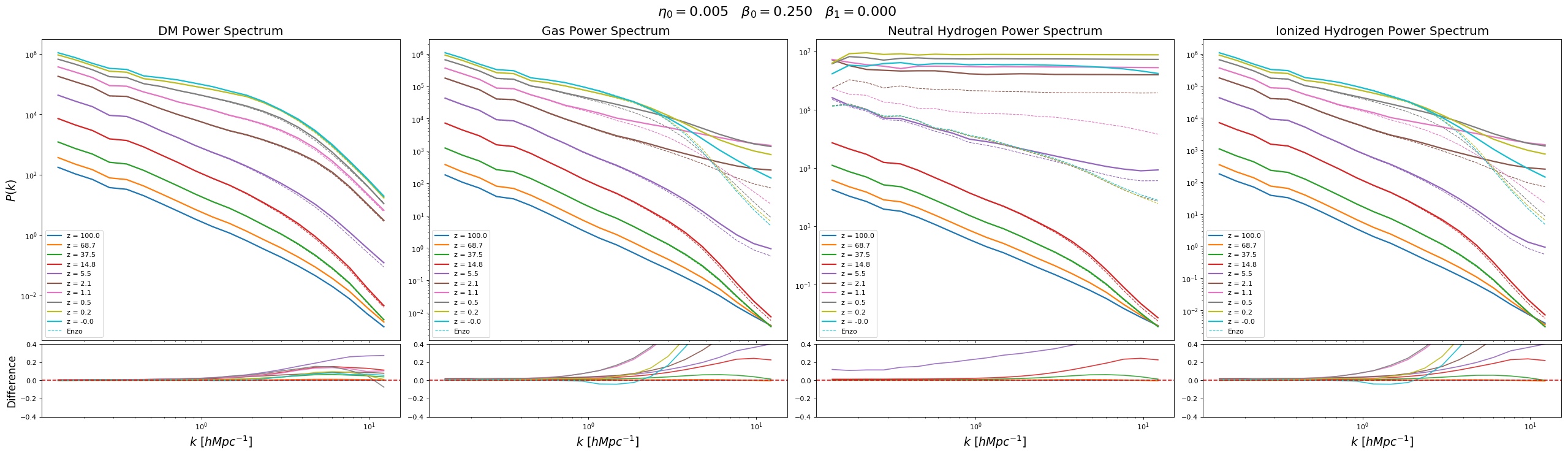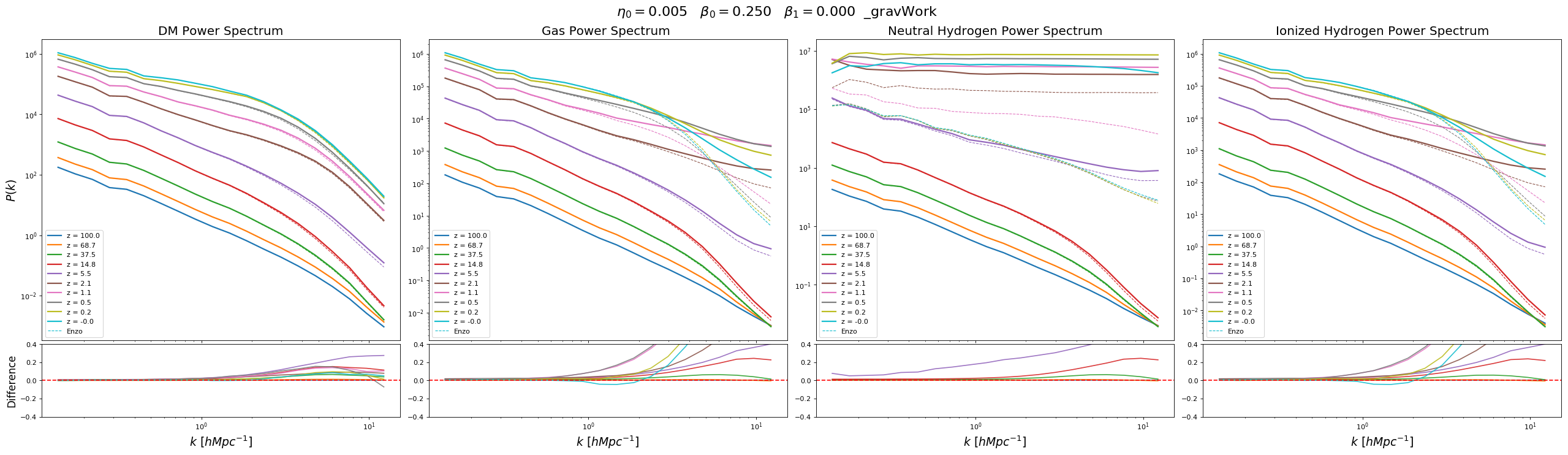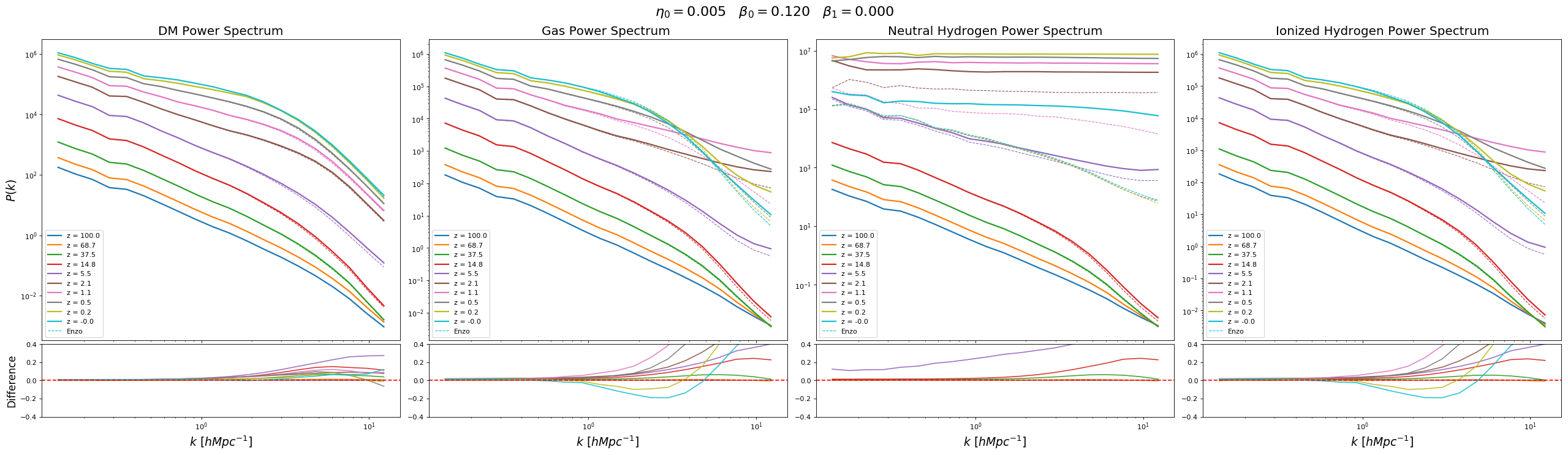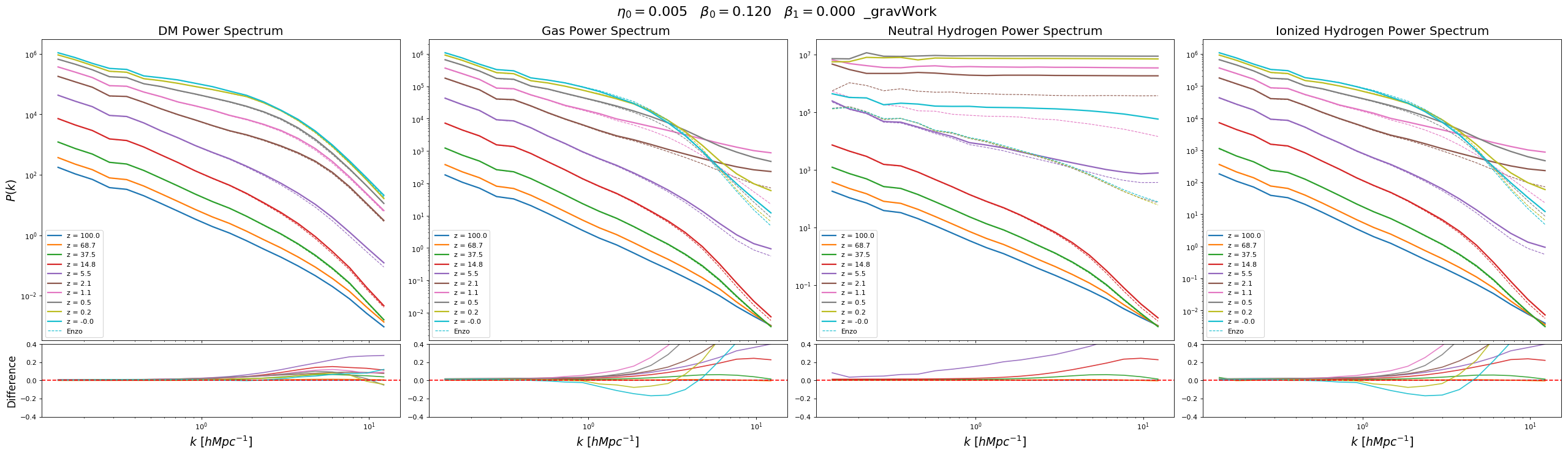Comparison of the total energy evolution using the change in kinetic energy against the gravitational work

Dual Energy Parameters: $\eta=0.005$ $\beta_0 = 0.25$ $\beta_1 = 0.0$

### Phase Diagram

Row 1: Using Change in Kinetic Energy.

Row 2: Using Gravitational Work.

### Power Spectrum

Using Change in Kinetic Energy.Using Gravitational Work.## Different Dual Energy Parameters

Dual Energy Parameters: $\eta=0.005$ $\beta_0 = 0.12$ $\beta_1 = 0.0$

### Phase Diagram

Row 1: Using Change in Kinetic Energy.

Row 2: Using Gravitational Work.

### Power Spectrum

Using Change in Kinetic Energy.Using Gravitational Work.